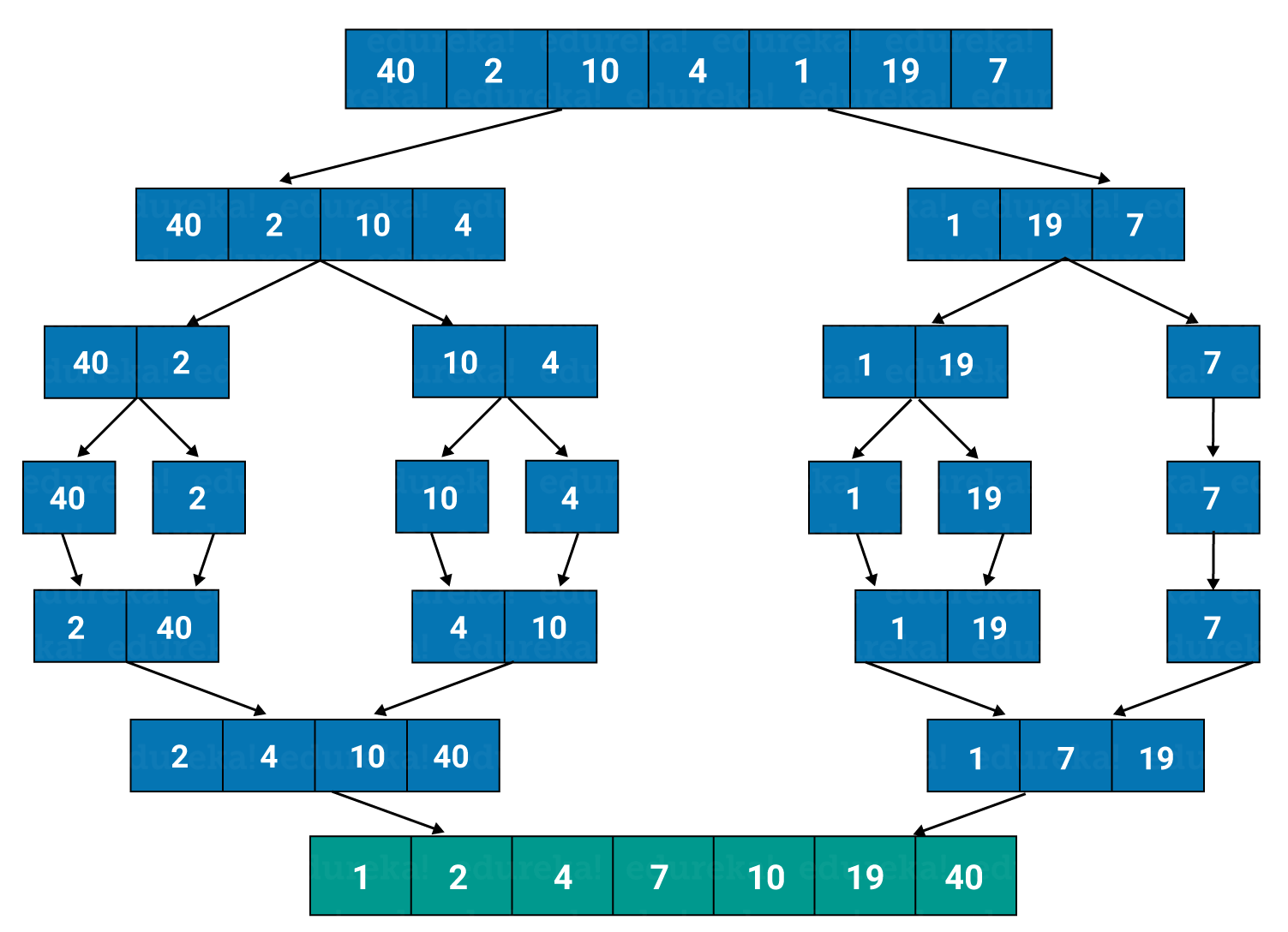# How to Perform Merge Sort in Java?13 / 29 Blog from Java Programs

Ever heard about the term, “Divide and Conquer”? This article is quite specifically based on this approach. Merge Sort is a “divide and conquer” algorithm where we first divide the problem into subproblems and then merge them together to conquer our solution. Here is a complete overview of the concept of merge sort in Java.

Let’s begin!

## What is merge sort in Java?

Merge sort is one of the popular sorting algorithms available and it follows a divide and conquer approach. A problem is divided into sub-problems and combined together to reach the final solution!

Now, what exactly happens during the working of merge sort? Let us understand in detail.

## Working of merge sort

There are two steps followed by the merge sort during the process:

• Divide: In this step, the input array is divided into 2 halves, the pivot is the midpoint of the array. This step is carried out recursively for all the half arrays until there are no more half arrays to divide further.
• Conquer: In this step, we sort and merge the divided arrays from bottom to top and reach towards our sorted array.

This approach helps you to easily sort the sub parts of the problems first and hence, reach the solution.

Let me show you a pictorial representation of merge sort.

## Example: DiagramHere, you saw how does a merge sort look like. The main concept of merge sort is that it takes less time to sort. Now, moving on towards our implementation part!

## Implementation

```package MyPackage;
public class MergeSort
{
void merge(int arr[], int beg, int mid, int end)
{
int l = mid - beg + 1;
int r = end - mid;
int LeftArray[] = new int [l];
int RightArray[] = new int [r];
for (int i=0; i<l; ++i)
LeftArray[i] = arr[beg + i];
for (int j=0; j<r; ++j)
RightArray[j] = arr[mid + 1+ j];
int i = 0, j = 0;
int k = beg;
while (i<l&&j<r)
{
if (LeftArray[i] <= RightArray[j])
{
arr[k] = LeftArray[i];
i++;
}
else
{
arr[k] = RightArray[j];
j++;
}
k++;
}
while (i<l)
{
arr[k] = LeftArray[i];
i++;
k++;
}
while (j<r)
{
arr[k] = RightArray[j];
j++;
k++;
}
}
void sort(int arr[], int beg, int end)
{
if (beg<end)
{
int mid = (beg+end)/2;
sort(arr, beg, mid);
sort(arr , mid+1, end);
merge(arr, beg, mid, end);
}
}
public static void main(String args[])
{
int arr[] = {40,51,22,45,1,4,90,23,17,55};
MergeSort ob = new MergeSort();
ob.sort(arr, 0, arr.length-1);
System.out.println("nSorted array");
for(int i =0; i<arr.length;i++)
{
System.out.println(arr[i]+"");
}
}
}```

Output:
Sorted array
1
4
17
22
23
40
45
51
55
90

This is how a Java code depicting merge sort looks like. Moving on towards the next segment.

## Complexity

Complexity is bifurcated into two types: Time complexity and Space complexity. In the case of merge sort, the data is as shown below:

 Complexity Best case Average Case Worst Case Time Complexity O(n log n) O(n log n) O(n log n) Space Complexity – – O(n)

With this, I shall conclude this article. I hope the contents explained above added value to your Java knowledge. We will keep exploring the Java world together. Stay tuned!

Check out the Java training by Edureka, a trusted online learning company with a network of more than 250,000 satisfied learners spread across the globe. Edureka’s Java J2EE and SOA training and certification course is designed for students and professionals who want to be a Java Developer. The course is designed to give you a head start into Java programming and train you for both core and advanced Java concepts along with various Java frameworks like Hibernate & Spring.

Got a question for us? Please mention it in the comments section of this “Merge sort in Java” blog and we will get back to you as soon as possible.

Upcoming Batches For Java Certification Training Course
Course NameDate
Java Certification Training Course

Class Starts on 15th July,2023

15th July

SAT&SUN (Weekend Batch)
View DetailsREGISTER FOR FREE WEBINARThank you for registering Join Edureka Meetup community for 100+ Free Webinars each month